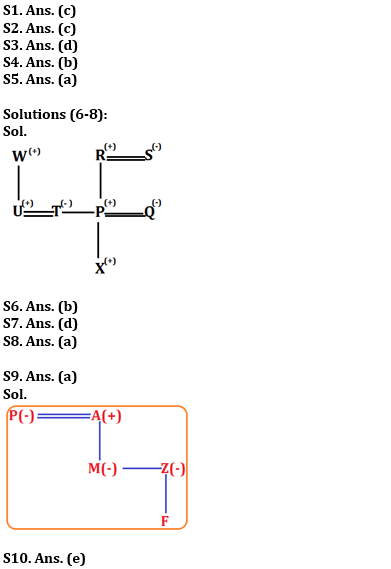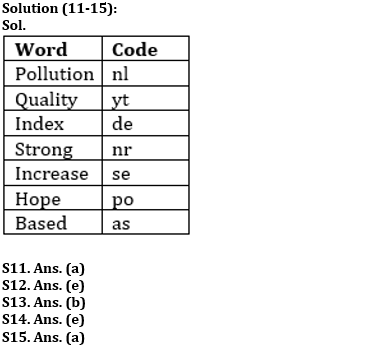Latest Banking jobs   »

# Reasoning Ability Quiz For IBPS RRB PO Prelims 2022- 4th June

Directions (1-5): Study the following sequence and answer the given questions.

A @ 3 % 4 E N M \$ 8 & 6 L D S ♠ 9 8 6 Q Y Z 1 7 % R O G ⧫ 2 I B 2 U &

Q1. Which of the following element is twelfth to the left of the twentieth element from the left end of the given arrangement?
(a) 6
(b) &
(c) M
(d) \$
(e) None of these

Q2. If all the symbols are dropped from the series, which element will be fourth to the right of the one which is twelfth from the right end?
(a) 9
(b) O
(c) R
(d) 7
(e) None of these

Q3. How many such numbers are there in the given series which are immediately preceded by a symbol and followed by a letter?
(a) None
(b) One
(c) Two
(d) Three
(e) Four

Q4. Four of the following five are alike in a certain way and forms a group, find the one that does not belong to that group?
(a) 3E%
(b) R⧫2
(c) M&\$
(d) D9S
(e) Y7Z

Q5. What should come in place of question mark (?) in the following series based on the above arrangement?
34%   N\$M   6DL   8Q6   ?
(a) %OR
(b) 7Z%
(c) O%R
(d) R%O
(e) R%7

Directions (6-8): Study the following information carefully to answer the given questions.
Eight Persons P, Q, R, S, T, U, W and X in a family. There are three married couple. T is sister P. R is grandfather of X. W is father of U. Q is daughter in law of S who is not married to W. X is unmarried male and T is married to U who has no child.

Q6. Who among the following is nephew of T?
(a) R
(b) X
(c) S
(d) Q
(e) None of these

Q7. Who among the following is son in law of S?
(a) P
(b) X
(c) R
(d) U
(e) None of these

Q8. What is the relation of Q with X?
(a) Mother
(b) Aunt
(c) Grand-Mother
(d) Sister
(e) Wife

Directions (9-10): Study the following information carefully and answer the questions which follow:
‘M + K’ means ‘M is brother of K’
‘M ÷ K’ means ‘M is father of K’
‘M × K’ means ‘M is wife of K’
‘M – K’ means ‘M is sister of K’
‘M = K’ means ‘M is mother of K’

Q9. In expression ‘P × A ÷ M – Z = F’, how is F related to P?
(a) Grandchild
(b) Grandson
(c) Grand daughter
(d) Grandmother
(e) Son

Q10.Which of the following expression represents the relationship ‘D is daughter – in – law of C’?
(a) D × H + N = E ÷ C
(b) H + D × N ÷ E = C
(c) C = F + L – Z × D
(d) C × F ÷ L ÷ Z × D
(e) None of these

Directions (11-15): Study the following information carefully and answer the questions given below:
In a certain code language,
‘Pollution Quality Index’ is written as ‘nl yt de’,
‘Strong increase hope’ is written as ‘po se nr’,
‘Quality Based Strong’ is written as ‘yt as nr’
‘Index Strong Hope’ is written as ‘nr de po’.

Q11. What is the code for ‘Pollution increase’ in the given code language?
(a) nl se
(b) nl po
(c) nr se
(d) de se
(e) None of these

Q12. What is the code for ‘Based’ in the given code language?
(a) po
(b) se
(c) nr
(d) yt
(e) None of these

Q13. What may be the code for ‘Strong opinion’ in the given code language?
(a) nr po
(b) nr fg
(c) se po
(d) nl de
(e) nr as

Q14. What is the code for ‘increase’ in the given code language?
(a) as
(b) nr
(c) po
(d) nl
(e) None of these

Q15. What may be the code for ‘Quality assured Index’?
(a) yt de dg
(b) nl yt as
(c) nr as yt
(d) de dg po
(e) po yt de

Solutions#### Congratulations!